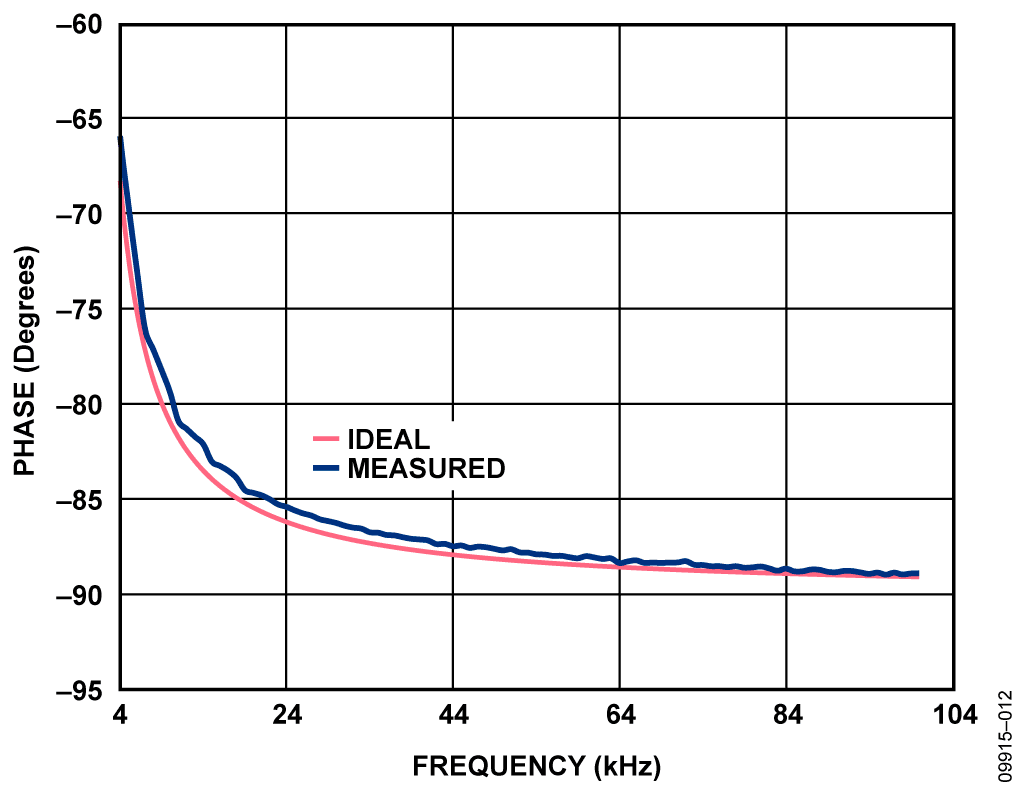## 设计资源

• 原理图
• 物料清单
• Gerber 文件
• 装配图

### 评估硬件

• EVAL-AD5933EBZ (\$60.77) High Accuracy Impedance Measurements Using 12-Bit Impedance Converters

## 优势和特点

• 高精度阻抗测量
• 采用内部频率发生器进行复杂的阻抗测量
• 最多可测量几百k-ohm

## 电路描述

 Range Output Excitation Amplitude Output Resistance, ROUT Range 1 1.98V p-p 200 Ω typ Range 2 0.97V p-p 2.4 kΩ typ Range 3 0.383V p-p 1.0 kΩ typ Range 4 0.198V p-p 600 Ω typ

 Range Output Excitation Amplitude Output DC Bias Level 1 1.98V p-p 1.48V 2 0.97V p-p 0.76V 3 0.383V p-p 0.31V 4 0.198V p-p 0.173V

## 电路评估与测试

 Parameter Value V p-p 1.98V (Range 1) Number of Settling Time Cycles 15 MCLK 16 MHz RCAL 20.1Ω RFB 20.0Ω Excitation Frequency Range 30 kHz to 30.2 kHz Unknown Impedances R1 = 10.3Ω, R2 = 30.0Ω, C3 = 1 µF (ZC = 5.3Ω< at 30 kHz)

 Parameter Value V p-p 0.198V (Range 4) Number of Settling Time Cycles 15 MCLK 16 MHz RCAL 99.85 kΩ RFB 100 kΩ Excitation Frequency Range 30 kHz to 50 kHz Unknown Impedances R0 = 99.85 kΩ R1 = 29.88 kΩ R2 = 14.95 kΩ R3 = 8.21 kΩ R4 = 217.25 kΩ C5 = 150 pF (ZC = 26.5 kΩ at 40 kHz) C6 = 47pF (ZC = 84.6 kΩ at 40 kHz)图 8. ZC = 8.21 kΩ, RCAL = 99.85 kΩ图 9. ZC = 217.25 kΩ, RCAL = 99.85 kΩ

R||C型结构也通常用于测量，，采用1 kΩ的RCAL、10 kΩ的R和10 nF的C，在频率范围4 kHz至100 kHz内进行测量。图11和12所示曲线表示幅度和相位结果和理想值的关系。

 Parameter Value V p-p 0.383V (Range 3) Number of Settling Time Cycles 15 MCLK 16 MHz RCAL 1 kΩ RFB 1 kΩ Excitation Frequency Range 4 kHz to 100 kHz Unknown Impedance R||C R = 10 kΩ C = 10 nF图11. ZC = 10 kΩ||10 nF, RCAL = 1 kΩ时的幅度结果图12. ZC = 10 kΩ||10 nF, RCAL = 1 kΩ时的相位结果

The evaluation software is that used on the EVAL-AD5933EBZ application board. Please refer to the technical note available on the CD provided for details on the board setup. Note that there are alterations to the schematic. Link connections on the EVAL-AD5933EBZ board are listed below in Table 4. Also note that the location for RFB is located at R3 on the evaluation board, and the location for ZUNKNOWN is C4.

Complete setup and operation for the hardware and software for the evaluation board can be found in User GuideUG-364.

 Link Number Default Position LK1 Open LK2 Open LK3 Insert LK4 Open LK5 Insert LK6 A